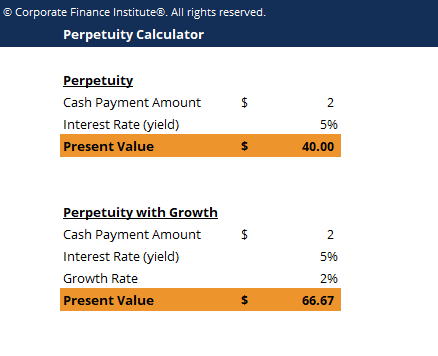# Perpetuity Calculator

## Perpetuity Calculator

This perpetuity calculator shows you how to compute present value of perpetuity and perpetuity with growth.

Below is a screenshot of the perpetuity calculator:### Download the Free Template

Enter your name and email in the form below and download the free template now!

### Perpetuity Calculator

Download the free Excel template now to advance your finance knowledge!

A perpetuity in the financial system is a situation where a stream of cash flow payments continues indefinitely or is an annuity which has no end. In valuation analysis, perpetuities are used to find the present value of a company’s future projected cash flow stream and company’s terminal value. Essentially, a perpetuity is a series of cash flows that keep paying out forever.

Although the total value of a perpetuity is infinite, it has a limited present value. The present value of an infinite stream of cash flow is calculated by adding up the discounted values of each annuity and the decrease of the discounted annuity value in each period until it reaches close to zero. The finite present value of a perpetuity is used by an analyst to determine the exact value of the company if it continues to perform at the same rate.

### Present value of perpetuity formula

PV = C / R

Where:

PV = Present value

C = Amount of continuous cash payment

r = Interest rate or yield

### Perpetuity with growth formula

PV = C / (r – g)

Where:

PV = Present value

C = Amount of continuous cash payment

r = Interest rate or yield

r = Growth Rate

### More Free Templates

For more resources, check out our business templates library to download numerous free Excel modeling, PowerPoint presentation and Word document templates.

• Excel Modeling Templates
• PowerPoint Presentation Templates
• Transaction Document Templates

### Financial Analyst Certification

Become a certified Financial Modeling and Valuation Analyst (FMVA)® by completing CFI’s online financial modeling classes and training program!